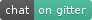349

39

53

30

# Lazy.jl``````Pkg.add("Lazy")
``````

Lazy.jl provides Julia with the cornerstones of functional programming - lazily-evaluated lists and a large library of functions for working with them. It's also a repository for some neat macros, which might be useful to you even if you don't want lazy lists (see below).

Firstly, the canonical examples, in Julia:

``````using Lazy

# Note : prepends. Don't forget the semicolon!
#   -- When running interactively, the semi-colon prevents the environment
#      from trying to display an infinite list.

# Fibonacci sequence defined in terms of itself:
fibs = @lazy 0:1:(fibs + drop(1, fibs));

take(20, fibs)
#> (0 1 1 2 3 5 8 13 21 34 55 89 144 233 377 610 987 1597 2584 4181)

# isprime defined in terms of the prime numbers:
isprime(n) =
@>> primes begin
takewhile(x -> x<=sqrt(n))
map(x -> n % x == 0)
any; !
end

# the prime numbers defined in terms of isprime:
primes = filter(isprime, Lazy.range(2));

take(20, primes)
#> (2 3 5 7 11 13 17 19 23 29 31 37 41 43 47 53 59 61 67 71)
``````

If you've done any functional programming, you already know how to use Lazy.jl; just head down to the reference below to see what functions are available.

### Intro to Laziness

For the unfamiliar, laziness just means that the elements of the list aren't actually calculated until you use them. This allows you to perform all sorts of magic, like working with infinite lists or lists of items from the future.

``````# Even square numbers:
> esquares = @>> Lazy.range() map(x->x^2) filter(iseven);
# first 5
> take(5, esquares)
List:
4
16
36
64
100
# 99th
> esquares
39204
``````

But lazy lists aren't just for mathematical tricks; you can use them very practically for things like file IO. For example, you might represent the lines of a terabyte-large file with a lazy list; you can process the lines as any other list, letting the IO happen in the background.

``````# TODO: lineseq example
@>> "file.txt" lineseq foreach(println) # Will work no matter many lines file.txt has
``````

The other thing that separates lists from arrays is the huge amount of functionality that comes with most functional programming libraries, including Lazy.jl - if you know your way around them, most data manipulation becomes a simple case of chaining a few functions together. Even if you do ultimately need arrays for speed, you could do worse than to prototype with lists.

### Macros

The threading macros will pipe values through functions, a bit like the `|>` operator but far more flexible. They can make code a lot cleaner by putting function calls in the order they are applied. The best way to understand them is by example:

``````# Just like x |> f etc.
@> x f == f(x)
@> x g f == f(g(x))
@> x a b c d e == e(d(c(b(a(x)))))

# Unlike |>, functions can have arguments - the value
# preceding a function will be treated as its first argument
@> x g(y, z) f == f(g(x, y, z))

@> x g f(y, z) == f(g(x), y, z)

# @>> does the exact same thing, but with value treated as the *last* argument.

@>> x g(y, z) f == f(g(y, z, x))

@>> x g f(y, z) == f(y, z, g(x))

# @as lets you name the threaded argument
@as x start_value f(x, y) g(z, x) == g(z, f(start_value, y))

# All threading macros work over begin blocks

@as x 2 begin
x^2
x+2
end == 6
``````

### Function Reference

``````List # The abstract type that represents lazy lists

list(1,2,3) == (1 2 3)

prepend(1, list(2,3,4)) == 1:list(2,3,4) == (1 2 3 4)

# Most list handling functions have similar names
# to those in Clojure.

# Create a seq from any iterator or array
seq([1,2,3]) == seq(1:3) == (1 2 3)

# Infinite list of an element
constantly(x) == (x x x ...)
constantly(1) == (1 1 1 ...)

# Infinite list of function calls
repeatedly(f) == (f() f() f() ...)
repeatedly(rand) == (0.634 0.478 0.776 ...)

# Infinitely repeat list
cycle(a) == (a... a... a... ...)
cycle([1,2,3]) == (1 2 3 1 2 3 1 2 3 1 ...)

# Repeatedly nest function calls
iterated(f, x) == (x f(x) f(f(x)) ...)
iterated(x->x^2, 2) == (2 4 16 256 65536 ...)

range(2) == (2 3 4 5 ...)
range(1, 5) == (1 2 3 4 5)
range(1, 5, 2) == (1 3 5)

list(1,2,3) * list(4,5,6) == (1 2 3 4 5 6)

first(list(1,2,3)) == 1
tail(list(1,2,3)) == (2 3)

flatten(list(1,2,list(3,4))) == (1 2 3 4)

takeuntil(x -> x > 1, 0:1) == (0 1)
takeuntil(x -> x > 1, 0:5) == (0 1 2)
takeuntil(x -> x > 1, 2:5) == (2)
takeuntil(x -> x > 1, []) == ()

riffle
interpose
take
drop
takelast
droplast
takenth
takewhile
dropwhile
# These work as for arrays, but are
# lazy where appropriate.
map, reduce, filter, reverse
lazymap
reductions
remove
dorun
foreach
distinct
groupby
partition
partitionby
splitat
splitby

# @lazy is the secret sauce that makes infinite definitions
# work; usually you can just wrap your list definition in it:
@lazy [1,2,3] == (1 2 3)
# Define a lazy recursive function
constantly(x) = @lazy x:constantly(x)

# Make a function map itself over lists
@listable exp
exp(range()) == (2.71 7.38 20.08 54.59 148.41 ...)

@>, @>>
``````

12/14/2013

7 days ago

268 commits